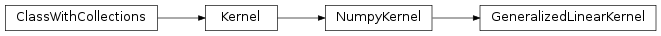# mvpa2.clfs.warehouse.GeneralizedLinearKernel¶class mvpa2.clfs.warehouse.GeneralizedLinearKernel(*args, **kwargs)

The linear kernel class.

Notes

Available conditional attributes:

• gradients: Dictionary of gradients per a parameter
• gradientslog: Dictionary of gradients per a parameter in logspace

(Conditional attributes enabled by default suffixed with +)

Attributes

 descr Description of the object if any

Methods

 add_conversion(typename, methodfull, methodraw) Adds methods to the Kernel class for new conversions as_ls(kernel) as_np() Converts this kernel to a Numpy-based representation as_raw_ls(kernel) as_raw_np() Directly return this kernel as a numpy array. as_raw_sg(kernel) Converts directly to a Shogun kernel as_sg(kernel) Converts this kernel to a Shogun-based representation cleanup() Wipe out internal representation compute(ds1[, ds2]) Generic computation of any kernel computed(\*args, \*\*kwargs) Compute kernel and return self reset()

Initialize instance of GeneralizedLinearKernel

Parameters: sigma_0 A simple constant squared value which is broadcasted across kernel. In the case of GPR – standard deviation of the Gaussian prior probability Normal(0, sigma_0**2) of the intercept of the linear regression. [Default: 1.0] Sigma_p A generic scalar or vector, or diagonal matrix to scale all dimensions or associate different scaling to each dimensions while computing te kernel matrix: k(x_A,x_B) = x_A^\top \Sigma_p x_B + \sigma_0^2. In the case of GPR – a scalar or a diagonal of covariance matrix of the Gaussian prior probability Normal(0, Sigma_p) on the weights of the linear regression. [Default: 1.0] enable_ca : None or list of str Names of the conditional attributes which should be enabled in addition to the default ones disable_ca : None or list of str Names of the conditional attributes which should be disabled

Attributes

 descr Description of the object if any

Methods

 add_conversion(typename, methodfull, methodraw) Adds methods to the Kernel class for new conversions as_ls(kernel) as_np() Converts this kernel to a Numpy-based representation as_raw_ls(kernel) as_raw_np() Directly return this kernel as a numpy array. as_raw_sg(kernel) Converts directly to a Shogun kernel as_sg(kernel) Converts this kernel to a Shogun-based representation cleanup() Wipe out internal representation compute(ds1[, ds2]) Generic computation of any kernel computed(\*args, \*\*kwargs) Compute kernel and return self reset()
reset()